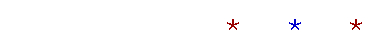Proyecto Salón Hogar

English SectionMath Page

# PRECALCULUS

Home

Internet Explorer 6 is the browser to view these pages, and Garamond is the font.

What is a rational number? Which numbers have rational square roots?  The decimal representation of irrationals. What is a real number?13.  Functions

What is a function? Functional notation. A function of a function.

The graph of a function. Coördinate pairs of a function. The height of the curve at x.

15.  Basic graphs

The constant function. The identity function. The absolute value function. A parabola. The square root function. The cubic function.

Definition of a polynomial in x. The degree of a term and of a polynomial. The leading coefficient. The general form of a polynomial.

The polynomial equation. The roots of a polynomial. The x- and y-intercepts of a graph. The relationship between the roots and the x-intercepts.

Definition of the slope. Positive and negative slope. A straight line has only one slope.
"Same slope" and "parallel." Perpendicular lines.
The slope and one point specify a straight line.

The equation of the first degree. The graph of a first degree equation -- a straight line. The slope-intercept form, and its proof.

Solving a quadratic equation by factoring. A double root. Quadratic inequalities. The sum and product of the roots.

Solving a quadratic equation by completing the square. The quadratic formula.

The remainder theorem.

The factor theorem. The fundamental theorem of algebra. The integer root theorem. Conjugate pairs.

Concave upward, concave downward.

Reflection about the x-axis. Reflection about the y-axis. Reflection through the origin.

Symmetry with respect to the y-axis. Symmetry with respect to the origin. Test for symmetry. Odd and even functions.

Definition of a translation. The equation of a circle.
The vertex of a parabola. Vertical stretches and shrinks.

Singularities. The reciprocal function. Horizontal and vertical asymptotes.Definition of inverses. Constructing the inverse.
The graph of an inverse function.

20.  Logarithms

The system of common logarithms. The system of natural logarithms. The three laws of logarithms.22.  Factorials

The Fundamental Principle of Counting.  Factorial representations.

Pascal's triangle.

A proof of the binomial theorem.Home

www.proyectosalonhogar.com